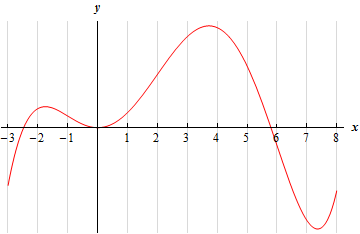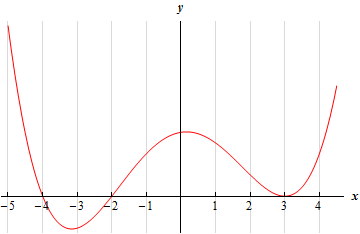Paul's Online Notes
Home / Calculus I / Applications of Derivatives / The Shape of a Graph, Part II
Show Mobile Notice Show All Notes Hide All Notes
Mobile Notice
You appear to be on a device with a "narrow" screen width (i.e. you are probably on a mobile phone). Due to the nature of the mathematics on this site it is best views in landscape mode. If your device is not in landscape mode many of the equations will run off the side of your device (should be able to scroll to see them) and some of the menu items will be cut off due to the narrow screen width.

### Section 4.6 : The Shape of a Graph, Part II

Section 1-1 : The Shape of a Graph, Part I
1. The graph of a function is given below. Determine the intervals on which the function is concave up and concave down.Solution
2. Below is the graph the 2nd derivative of a function. From this graph determine the intervals in which the function is concave up and concave down.Solution

For problems 3 – 8 answer each of the following.

1. Determine a list of possible inflection points for the function.
2. Determine the intervals on which the function is concave up and concave down.
3. Determine the inflection points of the function.

1. $$f\left( x \right) = 12 + 6{x^2} - {x^3}$$ Solution
2. $$g\left( z \right) = {z^4} - 12{z^3} + 84z + 4$$ Solution
3. $$h\left( t \right) = {t^4} + 12{t^3} + 6{t^2} - 36t + 2$$ Solution
4. $$h\left( w \right) = 8 - 5w + 2{w^2} - \cos \left( {3w} \right)$$ on $$\left[ { - 1,2} \right]$$ Solution
5. $$R\left( z \right) = z{\left( {z + 4} \right)^{\,{\frac{2}{3}}}}$$ Solution
6. $$h\left( x \right) = {{\bf{e}}^{4 - {x^{\,2}}}}$$ Solution

For problems 9 – 14 answer each of the following.

1. Identify the critical points of the function.
2. Determine the intervals on which the function increases and decreases.
3. Classify the critical points as relative maximums, relative minimums or neither.
4. Determine the intervals on which the function is concave up and concave down.
5. Determine the inflection points of the function.
6. Use the information from steps (a)(e) to sketch the graph of the function.

1. $$g\left( t \right) = {t^5} - 5{t^4} + 8$$ Solution
2. $$f\left( x \right) = 5 - 8{x^3} - {x^4}$$ Solution
3. $$h\left( z \right) = {z^4} - 2{z^3} - 12{z^2}$$ Solution
4. $$Q\left( t \right) = 3t - 8\sin \left( {\frac{t}{2}}} \right$$ on $$\left[ { - 7,4} \right]$$ Solution
5. $$f\left( x \right) = {x^{\,{\frac{4}{3}}}}\left( {x - 2} \right)$$ Solution
6. $$P\left( w \right) = w{{\bf{e}}^{4w}}$$ Solution
7. Determine the minimum degree of a polynomial that has exactly one inflection point. Solution
8. Suppose that we know that $$f\left( x \right)$$ is a polynomial with critical points $$x = - 1$$, $$x = 2$$ and $$x = 6$$. If we also know that the 2nd derivative is $$f''\left( x \right) = - 3{x^2} + 14x - 4$$. If possible, classify each of the critical points as relative minimums, relative maximums. If it is not possible to classify the critical points clearly explain why they cannot be classified. Solution